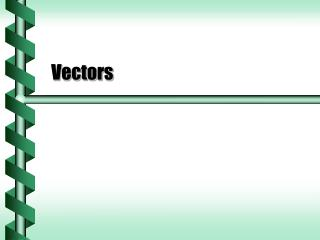DownloadDownload PresentationVectors

# Vectors

Download Presentation## Vectors

- - - - - - - - - - - - - - - - - - - - - - - - - - - E N D - - - - - - - - - - - - - - - - - - - - - - - - - - -
##### Presentation Transcript

1. Vectors

2. Scalars • Consider the following objects • Thermometer • Beaker • Battery tester • Clock • What do all these have in common?

3. Scalar Quantities • Any measurement that consists of a single number is a scalar. • 72 °F • 500 milliliters • 1.5 volts • 6 hours, 24 minutes (consider this as 6.4 h) • Most measured quantities consist of only a single magnitude.

4. Magnitude and Direction • Position, velocity and acceleration have both a magnitude and a direction. • It takes at least two measurements to describe these values. • Position on a map (N-S and E-W position) • These quantities can be thought of as carrying the value from a starting point to a destination. • The word vector means carrier.

5. Vector Quantities • A measurement that requires more than one value to describe it is a vector. • 80 km/h to the northwest • 5 paces east, 3 paces south, and dig down 2 feet • 200 m/s bearing 270° launched upwards at 30° • 9.8 m/s2 directed down • The values within a vector measurement don’t always have the same unit, but it should be possible to convert them into the same units.

6. One representation of a vector is an arrow. The tail shows the start of the vector. The tip points in the direction. The length of the arrow shows the magnitude. Vector Diagram tip: direction length: magnitude tail: start

7. Vector Notation • A vector variable is represented by a small arrow over the top of the variable. • Some texts use boldface for vectors, but that can be hard to distinguish on some backgrounds.

8. Vectors in Equations • Vector variables can be used in equations • For instance, • Vector variables are different from scalar variables • They are different dimension,

9. 2-D and 3-D Vectors • Some vectors have two dimensions. • Two directions on a horizontal surface (boat on a lake) • Vertical and one horizontal direction (ball tossed in air) • Full motion may require three dimensions. • Two horizontal and one vertical • (motion of a plane circling while landing) next